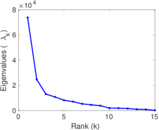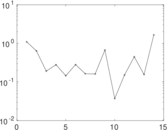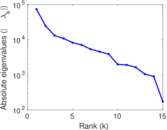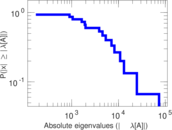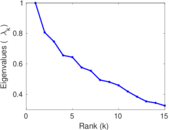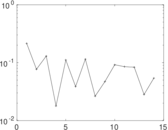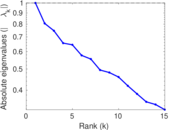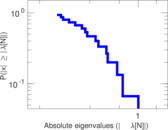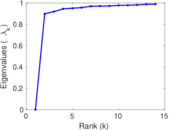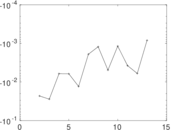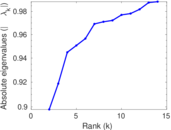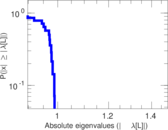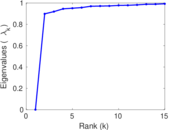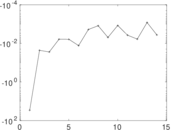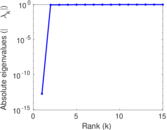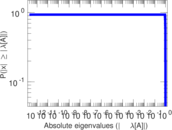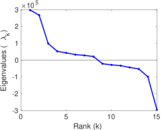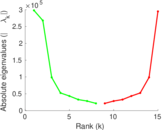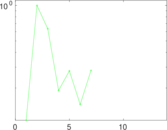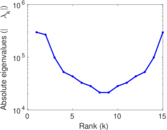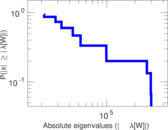# Discogs label–genre

Discogs (short for "discographies") is a large online music database that provides information about audio records including information about artists, labels and release details. Each edge of this bipartite network connects a label and a genre. An edge indicates that the label was involved in the production of a release of this genre. The left nodes represent labels and the right nodes represent genres.

 Code `Dr` Internal name `discogs_lgenre` Name Discogs label–genre Data source http://www.discogs.com/ AvailabilityDataset is available for download Consistency checkDataset passed all tests Category Feature network Node meaning Label, genre Edge meaning Feature Network formatBipartite, undirected Edge typeUnweighted, multiple edges

## Statistics

 Size n = 270,786 Left size n1 = 270,771 Right size n2 = 15 Volume m = 4,147,665 Unique edge count m̿ = 481,661 Wedge count s = 18,190,840,285 Claw count z = 647,168,119,285,166 Cross count x = 1.913 97 × 1019 Square count q = 2,880,892,476 4-Tour count T4 = 95,812,257,190 Maximum degree dmax = 1,517,976 Maximum left degree d1max = 78,434 Maximum right degree d2max = 1,517,976 Average degree d = 30.634 3 Average left degree d1 = 15.318 0 Average right degree d2 = 276,511 Fill p = 0.118 590 Average edge multiplicity m̃ = 8.611 17 Size of LCC N = 270,785 Diameter δ = 4 50-Percentile effective diameter δ0.5 = 3.039 86 90-Percentile effective diameter δ0.9 = 3.807 97 Median distance δM = 4 Mean distance δm = 3.041 54 Gini coefficient G = 0.923 545 Balanced inequality ratio P = 0.097 669 0 Left balanced inequality ratio P1 = 0.148 640 Right balanced inequality ratio P2 = 0.226 562 Relative edge distribution entropy Her = 0.632 470 Power law exponent γ = 3.698 21 Tail power law exponent γt = 3.111 00 Tail power law exponent with p γ3 = 3.111 00 p-value p = 0.000 00 Left tail power law exponent with p γ3,1 = 3.121 00 Left p-value p1 = 0.000 00 Right tail power law exponent with p γ3,2 = 6.771 00 Right p-value p2 = 0.000 00 Degree assortativity ρ = −0.351 885 Degree assortativity p-value pρ = 0.000 00 Spectral norm α = 73,782.4 Algebraic connectivity a = 0.897 895 Spectral separation |λ1[A] / λ2[A]| = 2.985 07 Controllability C = 270,756 Relative controllability Cr = 0.999 889

## Plots

### Degree distribution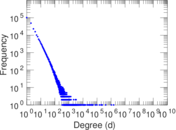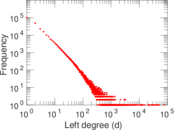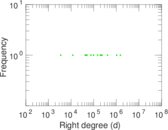### Cumulative degree distribution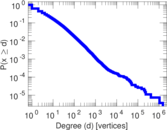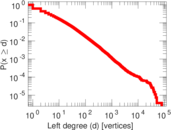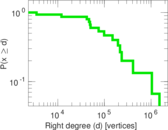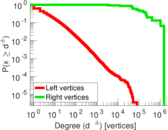### Lorenz curve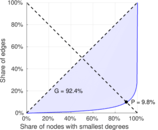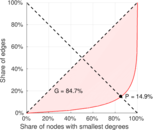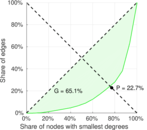### Spectral distribution of the adjacency matrix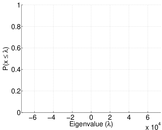### Spectral distribution of the normalized adjacency matrix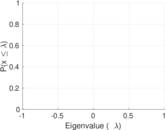### Spectral distribution of the Laplacian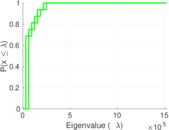### Spectral graph drawing based on the adjacency matrix### Spectral graph drawing based on the Laplacian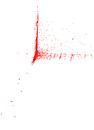### Spectral graph drawing based on the normalized adjacency matrix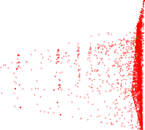### Degree assortativity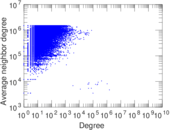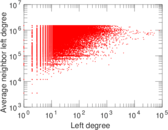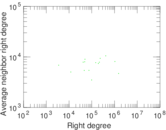### Zipf plot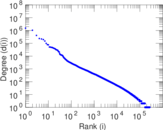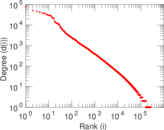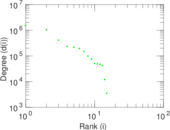### Hop distribution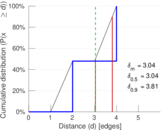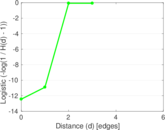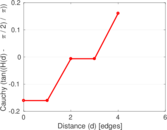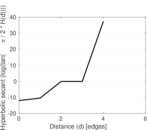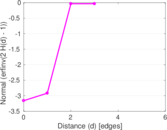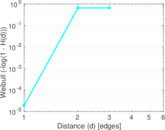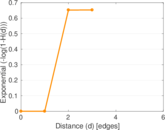### Delaunay graph drawing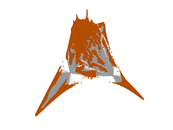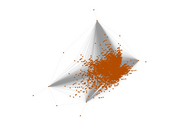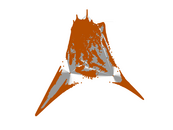### Edge weight/multiplicity distribution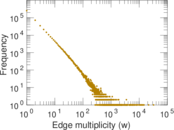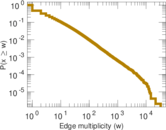### Matrix decompositions plots